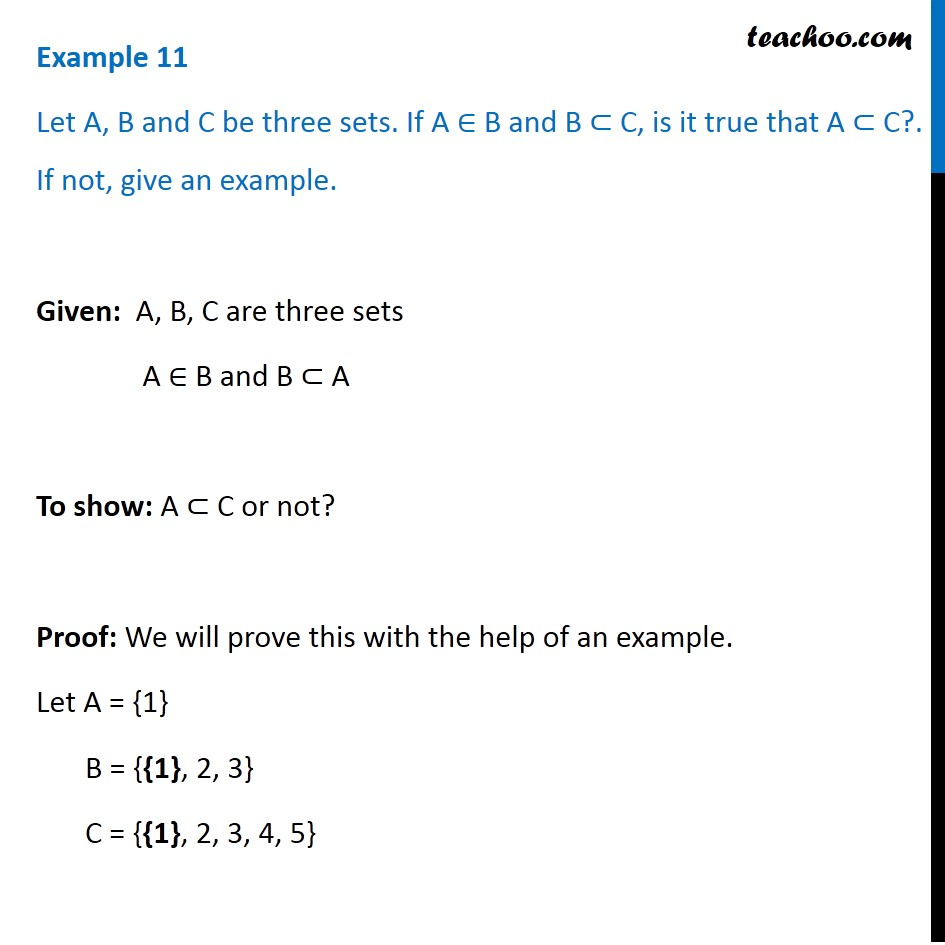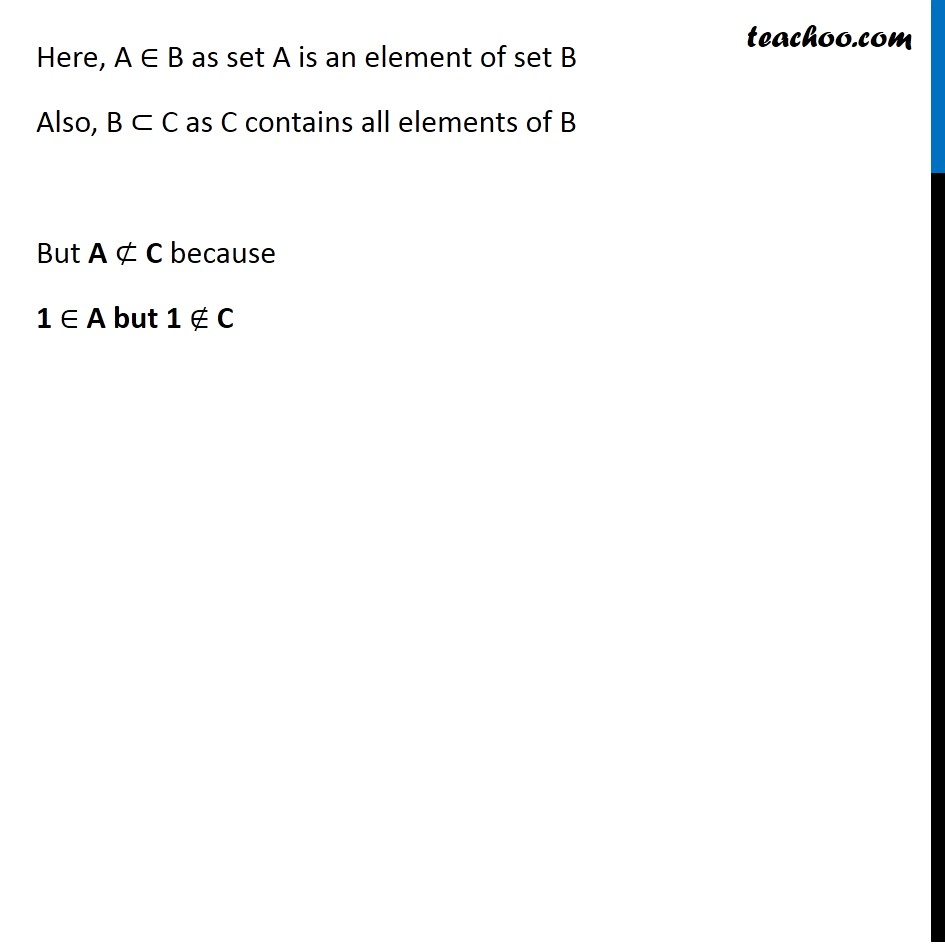Examples

Chapter 1 Class 11 Sets
Serial order wiseLearn in your speed, with individual attention - Teachoo Maths 1-on-1 Class

### Transcript

Example 11 Let A, B and C be three sets. If A ∈ B and B ⊂ C, is it true that A ⊂ C?. If not, give an example. Given: A, B, C are three sets A ∈ B and B ⊂ A To show: A ⊂ C or not? Proof: We will prove this with the help of an example. Let A = {1} B = {{1}, 2, 3} C = {{1}, 2, 3, 4, 5} Here, A ∈ B as set A is an element of set B Also, B ⊂ C as C contains all elements of B But A ⊄ C because 1 ∈ A but 1 ∉ C